Function Repository Resource:

# SteinerCircumellipse

Generate the Steiner circumellipse of a 2D triangle

Contributed by: Jan Mangaldan
 ResourceFunction["SteinerCircumellipse"][{p1,p2,p3}] returns an Ellipsoid representing the Steiner circumellipse of the triangle defined by vertices p1,p2 and p3. ResourceFunction["SteinerCircumellipse"][{p1,p2,p3},property] gives the value of the specified property.

## Details

The Steiner circumellipse of a triangle is the unique ellipse centered at the triangle's centroid that passes through all the triangle's vertices.
The following properties are supported:
 "Ellipsoid" Ellipsoid representing the circumellipse "Parametric" parametric equation for the circumellipse as a pure function "Implicit" implicit Cartesian equation for the circumellipse as a pure function
ResourceFunction["SteinerCircumellipse"][poly] where poly is a Triangle or Polygon is equivalent to ResourceFunction["SteinerCircumellipse"][PolygonCoordinates[poly]].

## Examples

### Basic Examples (1)

Show a triangle together with its Steiner circumellipse:

 In:=Out=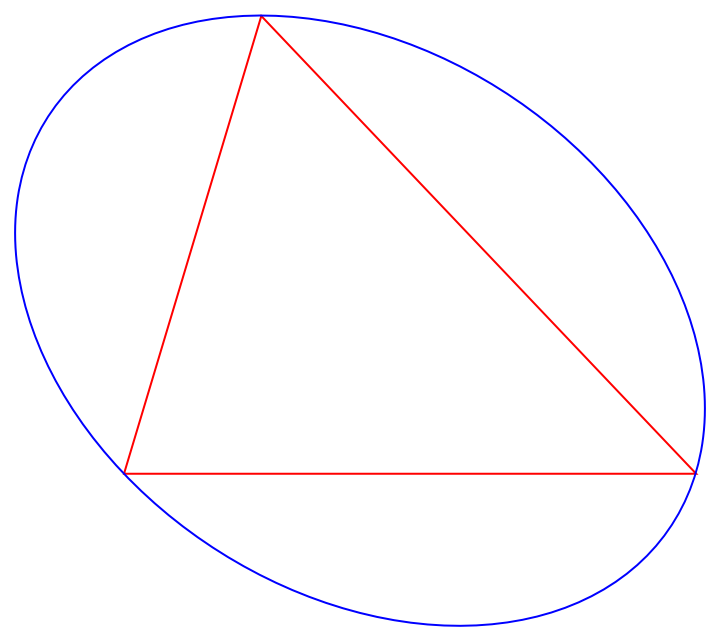### Scope (5)

A triangle:

 In:=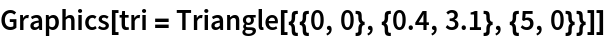Out=Generate the parametric equation of the triangle's Steiner circumellipse:

 In:=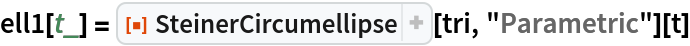Out=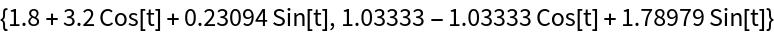Plot the parametric equation along with the triangle:

 In:=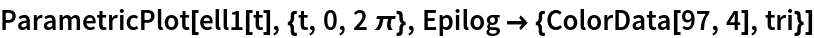Out=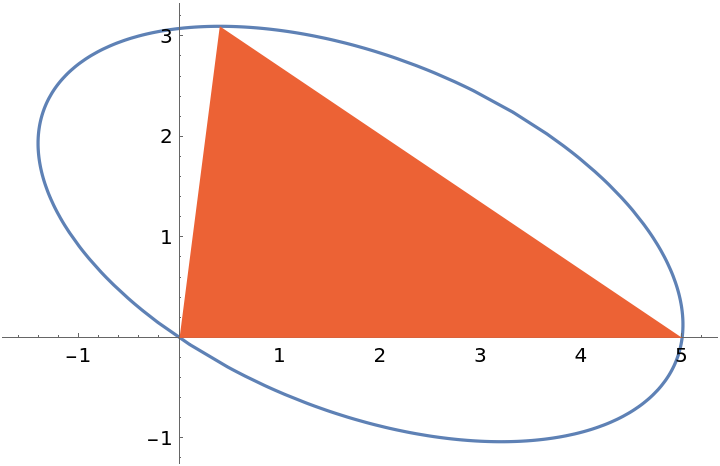Generate the implicit equation of the triangle's Steiner circumellipse:

 In:=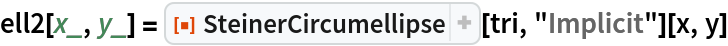Out=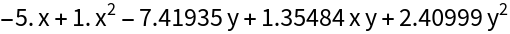Plot the implicit equation along with the triangle:

 In:=Out=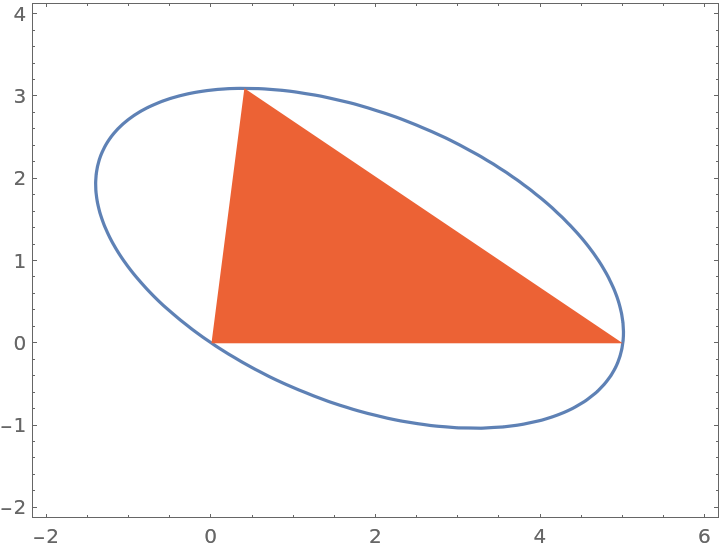### Properties and Relations (3)

Use the resource function EllipseProperties to generate properties of the circumellipse:

 In:=Out=The area of the Steiner circumellipse is a constant multiple of the area of the original triangle:

 In:=Out=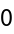The Steiner inellipse is the Steiner circumellipse scaled by a factor of 1/2. It passes through the midpoints of the triangle's sides:

 In:=Out=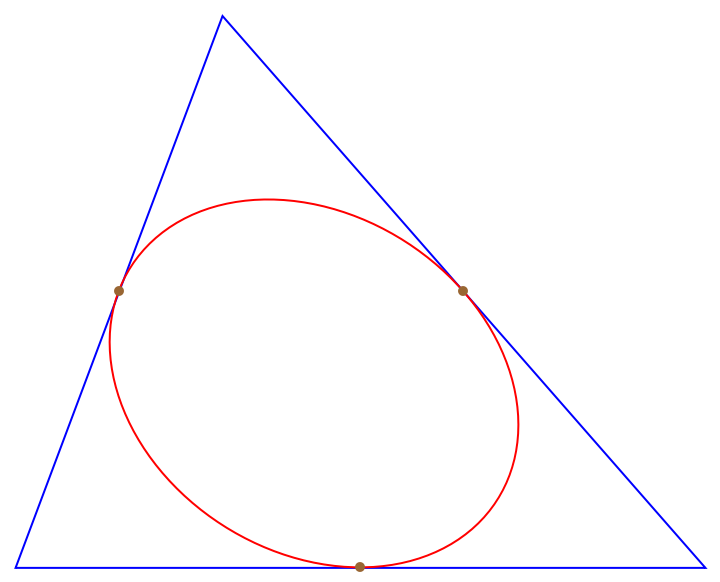### Neat Examples (1)

Visualize Marden's theorem for a cubic polynomial:

 In:=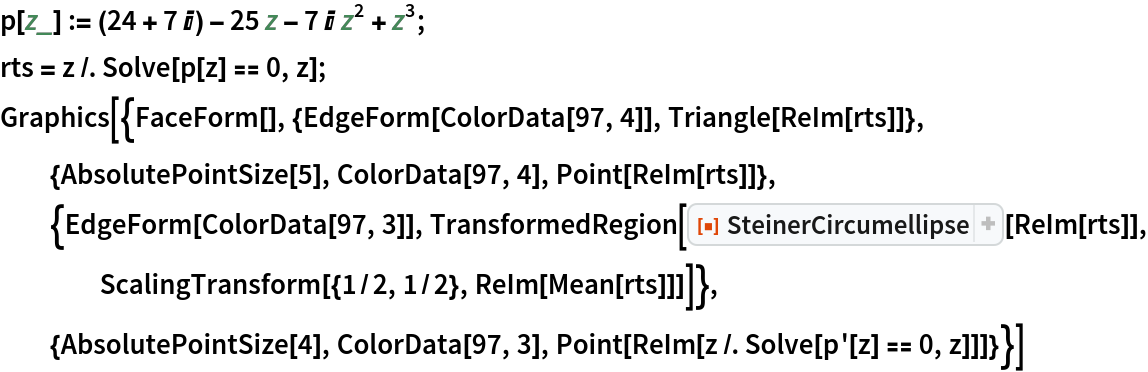Out=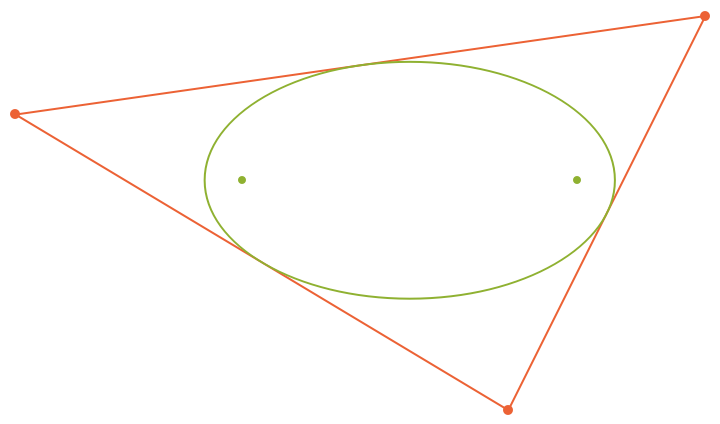## Version History

• 1.0.0 – 23 August 2021